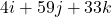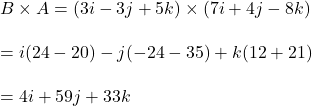## what is vector product B×A of two vectors A=7i+4j-8k and B=3i-3j+5k​

Question

what is vector product B×A of two vectors A=7i+4j-8k and B=3i-3j+5k​

in progress 0
5 months 2021-09-05T13:41:37+00:00 1 Answers 3 views 0

## Answers ( )Explanation:

Given that,

Vector A = 7i+4j-8k

vector B = 3i-3j+5k​

We need to find B×A. It can be calculated as follows :So, the value of vector product is.